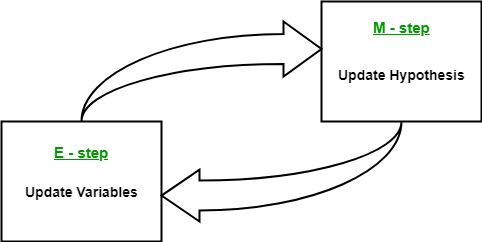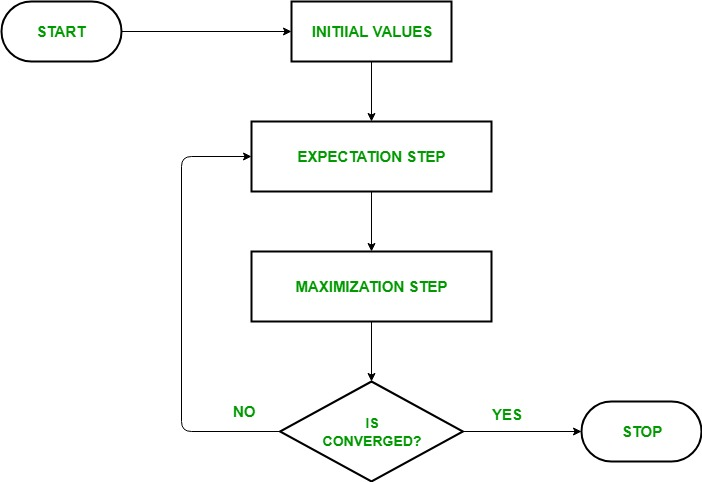Related Articles
ML | Expectation-Maximization Algorithm
• Difficulty Level : Easy
• Last Updated : 14 May, 2019

In the real-world applications of machine learning, it is very common that there are many relevant features available for learning but only a small subset of them are observable. So, for the variables which are sometimes observable and sometimes not, then we can use the instances when that variable is visible is observed for the purpose of learning and then predict its value in the instances when it is not observable.

On the other hand, Expectation-Maximization algorithm can be used for the latent variables (variables that are not directly observable and are actually inferred from the values of the other observed variables) too in order to predict their values with the condition that the general form of probability distribution governing those latent variables is known to us. This algorithm is actually at the base of many unsupervised clustering algorithms in the field of machine learning.
It was explained, proposed and given its name in a paper published in 1977 by Arthur Dempster, Nan Laird, and Donald Rubin. It is used to find the local maximum likelihood parameters of a statistical model in the cases where latent variables are involved and the data is missing or incomplete.

Algorithm:

1. Given a set of incomplete data, consider a set of starting parameters.
2. Expectation step (E – step): Using the observed available data of the dataset, estimate (guess) the values of the missing data.
3. Maximization step (M – step): Complete data generated after the expectation (E) step is used in order to update the parameters.
4. Repeat step 2 and step 3 until convergence.The essence of Expectation-Maximization algorithm is to use the available observed data of the dataset to estimate the missing data and then using that data to update the values of the parameters. Let us understand the EM algorithm in detail.

• Initially, a set of initial values of the parameters are considered. A set of incomplete observed data is given to the system with the assumption that the observed data comes from a specific model.
• The next step is known as “Expectation” – step or E-step. In this step, we use the observed data in order to estimate or guess the values of the missing or incomplete data. It is basically used to update the variables.
• The next step is known as “Maximization”-step or M-step. In this step, we use the complete data generated in the preceding “Expectation” – step in order to update the values of the parameters. It is basically used to update the hypothesis.
• Now, in the fourth step, it is checked whether the values are converging or not, if yes, then stop otherwise repeat step-2 and step-3 i.e. “Expectation” – step and “Maximization” – step until the convergence occurs.

Flow chart for EM algorithm –Usage of EM algorithm –

• It can be used to fill the missing data in a sample.
• It can be used as the basis of unsupervised learning of clusters.
• It can be used for the purpose of estimating the parameters of Hidden Markov Model (HMM).
• It can be used for discovering the values of latent variables.

• It is always guaranteed that likelihood will increase with each iteration.
• The E-step and M-step are often pretty easy for many problems in terms of implementation.
• Solutions to the M-steps often exist in the closed form.